# Introduction to KDB.AI (2) - Sample code (document search)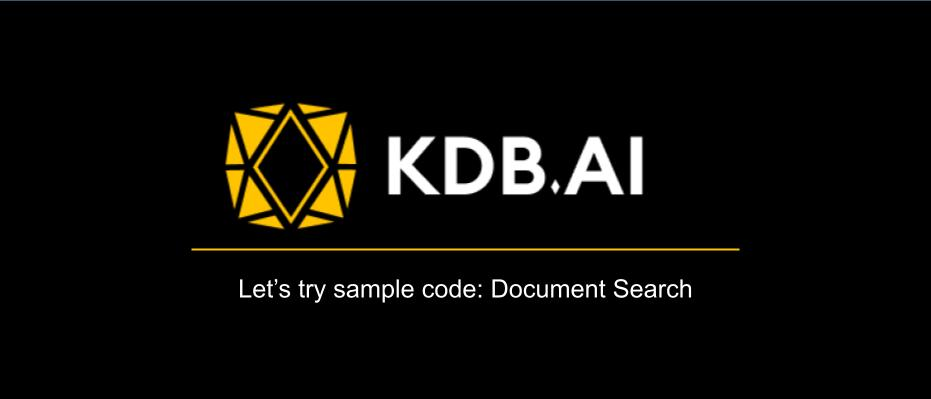### Introduction

This is Jung from the Lakehouse Department of the GLB Division.

In this article, I would like to introduce the sample code practice of KDB.AI that I introduced in the previous article.

KDB.AI is a Vector database powered by kdb+, the world's fastest time series database and analysis engine, and can be connected via Endpoint and API Key.

The Early Access Program was recently launched and you can try it out with a free account.

Instructions on how to create an account and Endpoints & API Keys can be found in the previous article.techblog.ap-com.co.jp

Please create an account, Endpoints, and API Keys to practice the sample code.

This time, we will introduce Document Search as a sample.

The order of the articles is an introduction to Document Search, followed by a sample code exercise.

### Contents

Document Search is a sample that uses KDB.AI as a Vector Store to search the contents of PDF.

We will practice performing semantic search on unstructured text PDF. Semantic search is a technology that understands the search query that the user is interested in and presents search results based on that understanding. Identify related results by finding similar vectors, even if the data are not exactly the same.

In the practical training, you will go through the following process.

➀ Read a PDF and split each text into sentences using Python's PyPDF2 and spacy libraries.

➁ Create a Sentence Transformers model, input the text sentences divided by ➀ into the model, and create Vector Embedding.

③ Create a table in KDB.AI and save the Embedding.

④ Put the content you are interested in into the model ➁ and embedding it.

⑤ Input the Vector from ④ into KDB.AI's search function to obtain similar results.

➝ In other words, use KDB.AI to perform a semantic search using Query.

### Sample code practice

The training was conducted using KDB.AI. kdb.ai

Let's try out the sample code on Databricks Workspace.

##### 0. Databricks Cluster

Cluster uses 14.0 ML (includes Apache Spark 3.5.0, Scala 2.12). The above Cluster is Python3.10.12 version.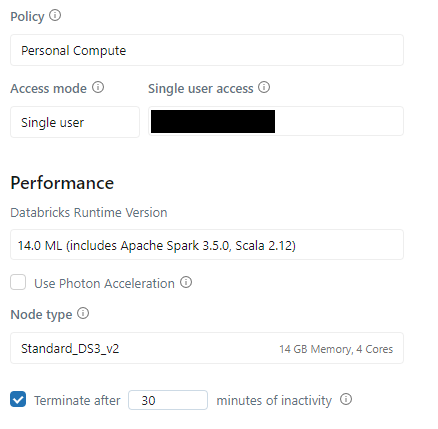Install the package to be able to read and split PDFs.

• Install PyPDF2 to handle PDF.
```%pip install PyPDF2 spacy sentence-transformers kdbai_client -q
```
• Install spaCy for Advanced Natural Language Processing.
```!python3 -m spacy download en_core_web_sm -q
```

Load a PDF and split it into sentences.

• Enter the path of the pdf in "My path where I uploaded the pdf".
```import PyPDF2
import spacy

# Create a function to split PDF into sentences
def split_pdf_into_sentences(pdf_path):
# Open PDF
with open(pdf_path, "rb") as pdf_file:

# Extract and combine text from each page
full_text = ""
full_text += page.extract_text()

# Tokenize text using spaCy
doc = nlp(full_text)
sentences = [sent.text for sent in doc.sents]

return sentences

# Define the path of the PDF to use
pdf_path = "
My path where I uploaded the pdf"

# Input the PDF into the created function
pdf_sentences = split_pdf_into_sentences(pdf_path)

# Number of sentences
len(pdf_sentences)
```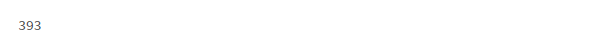Check the divided sentences.

```pdf_sentences
```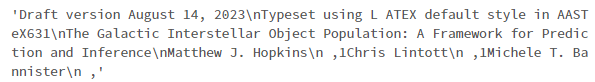##### 2. Creating Vector Embedding

Create embeddings for divided sentences using the Sentence Transformers library.

Select the Sentence Transformer model.

• In this sample, we will use the pre-trained model "all-MiniLM-L6-v2".
```from sentence_transformers import SentenceTransformer

model = SentenceTransformer("all-MiniLM-L6-v2")
```

Create an embedding.

• Input the divided sentences into the Sentence Transformer model and embedding them through encoding.
• Convert it to a DataFrame format that can be supported by KDB.AI.
```import numpy as np
import pandas as pd

# Create an embedding.
embeddings_array = model.encode(np.array(pdf_sentences))
embeddings_list = embeddings_array.tolist()
embeddings_df = pd.DataFrame({"vectors": embeddings_list, "sentences": pdf_sentences})
embeddings_df
```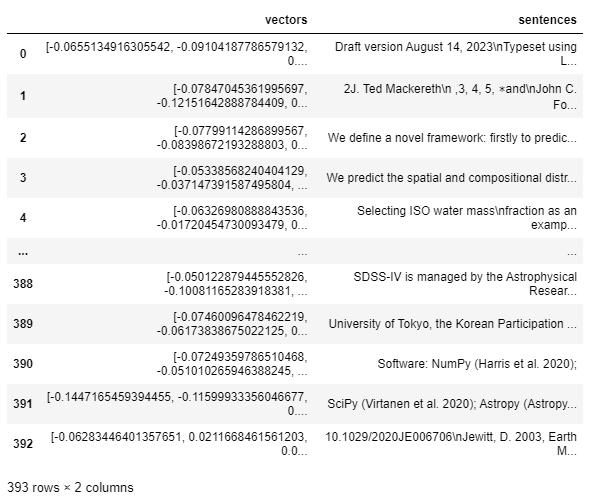• Embedding dimension is 384.
• The above dimension is planned to be used as the VectorIndex dimension when defining the schema of the KDB.AI table that stores the embedding.
```len(embeddings_df["vectors"])
```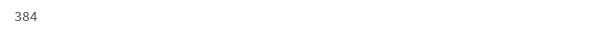##### 3. Save embedding to KDB.AI

Connect to KDB.AI Session using Endpoints and API Keys.

• Instructions on how to create Endpoints and API Keys can be found in the previous blog. techblog.ap-com.co.jp

• Enter your KDB.AI ENDPOINT in "My KDB.AI ENDPOINT".

• Enter your KDB.AI API KEY in "My KDB.AI API KEY".
```import kdbai_client as kdbai

KDBAI_ENDPOINT = "My KDB.AI ENDPOINT"
KDBAI_API_KEY = "My KDB.AI API KEY"
session = kdbai.Session(api_key=KDBAI_API_KEY, endpoint=KDBAI_ENDPOINT)
```

Define the schema of the KDB.AI table that stores the embedding.

• Select the index and metric to use for search.
• In this sample, we will use HNSW (Hierarchical Navigable Small World) as the index.
• HNSW is a method that searches for nearest neighbors, so it can efficiently process high-dimensional data such as text documents and natural language.
• The similarity metric uses L2 of Euclidean distance.
• Euclidean distance L2 calculates the distance of coordinates in high-dimensional space.
• Enter the dimension 384 confirmed above.

Please check the following page for details on the index handled by KDB.AI. code.kx.com

Please check the following page for details on the metrics handled by KDB.AI. code.kx.com

```pdf_schema = {
"columns": [
{"name": "sentences", "pytype": "str"},
{
"name": "vectors",
"vectorIndex": {"dims": 384, "metric": "L2", "type": "hnsw"},
},
]
}
```

Create a table.

• Create a pdf table with the session.create_table() function.
```table = session.create_table("pdf", pdf_schema)
```
• Check the table with the query() function.
• The table is still empty.
```table.query()
```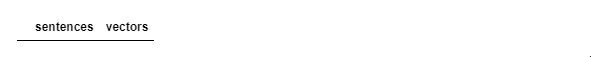Enter Embedding in the table.

```table.insert(embeddings_df)
```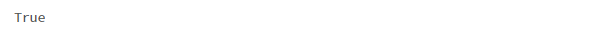• Also, data is added to the table by query().
```table.query()
```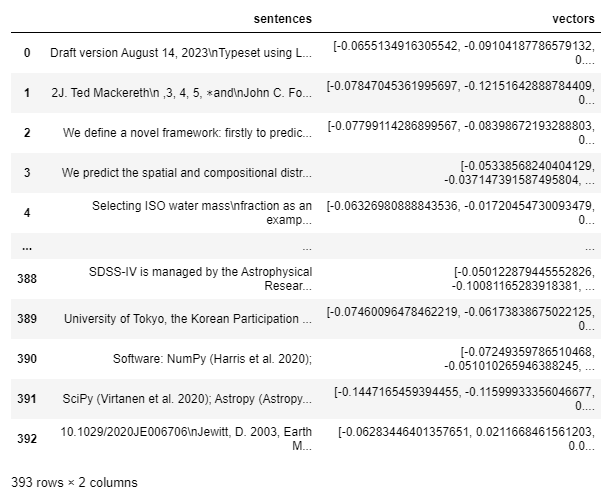##### 4. Query search using KDB.AI

Embedding is now saved in KDB.AI, so you can perform semantic search.

• Enter the search term you want to search into the Sentence Transformer model and embedding it through encoding.
• Search KDB.AI for the index created from the model.
• Returns the three most similar.
```search_term = "number of interstellar objects in the milky way"
search_term_vector = model.encode(search_term)
search_term_list = [search_term_vector.tolist()]

results = table.search(search_term_list, n=3)
results
```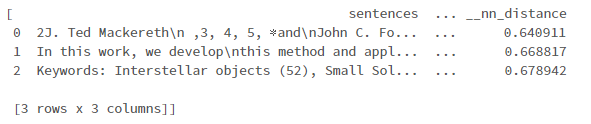• Find the three most similar sentences.
• Set the width of the displayed text using pd.set_option() and check the entire text.
```# Set to look at the entire column value instead of reducing it to...
pd.set_option("display.max_colwidth", None)
# Check the text
results["sentences"]
```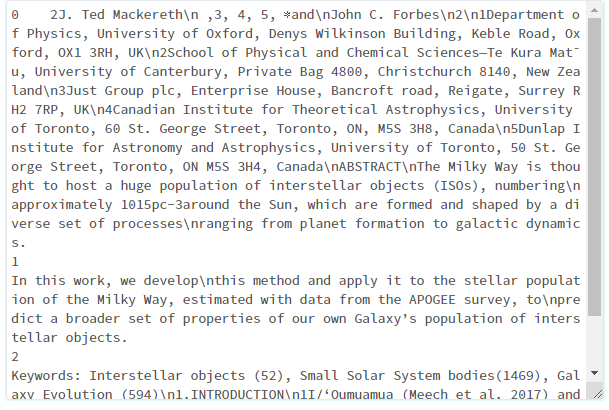• I'll try other searches as well.
```search_term = "how does planet formation occur"
search_term_vector = model.encode(search_term)
search_term_list = [search_term_vector.tolist()]

results = table.search(search_term_list, n=3)
results["sentences"]
```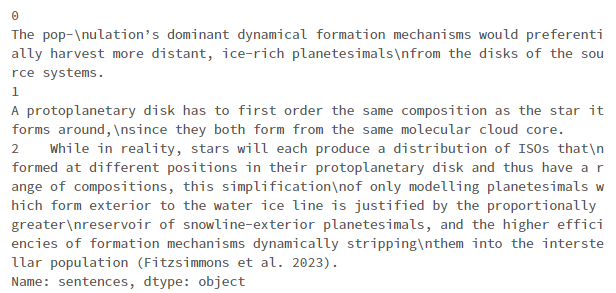##### 5. Delete table

Delete the table.

```table.drop()
```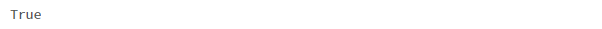### Summary

In this post, I introduced Document Search, a sample code for KDB.AI on Databricks, and ran the sample code on Databricks as an exercise.

Through the practical training, I learned the following:

• How to read PDF and split each text into sentences with PyPDF2 and spacy libraries
• How to create Vector Embedding through Sentence Transformers model
• How to perform a semantic search using Query using KDB.AI

In the next post, I would like to test other KDB.AI sample codes on Databricks and introduce them as in this post.

Thank you for reading until the end. Thank you for your continued support!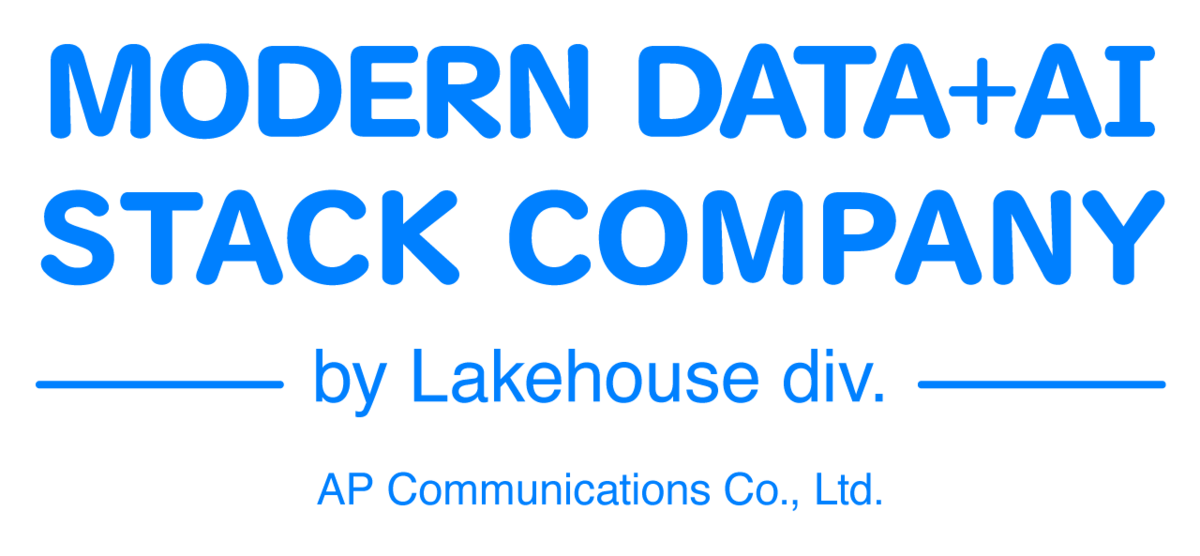We provide a wide range of support, from the introduction of data analysis platforms using Databricks to support for in-house production. If you are interested, please feel free to contact us.

We are also looking for people to work with us! We look forward to hearing from anyone interested in APC.

Translated by Johann